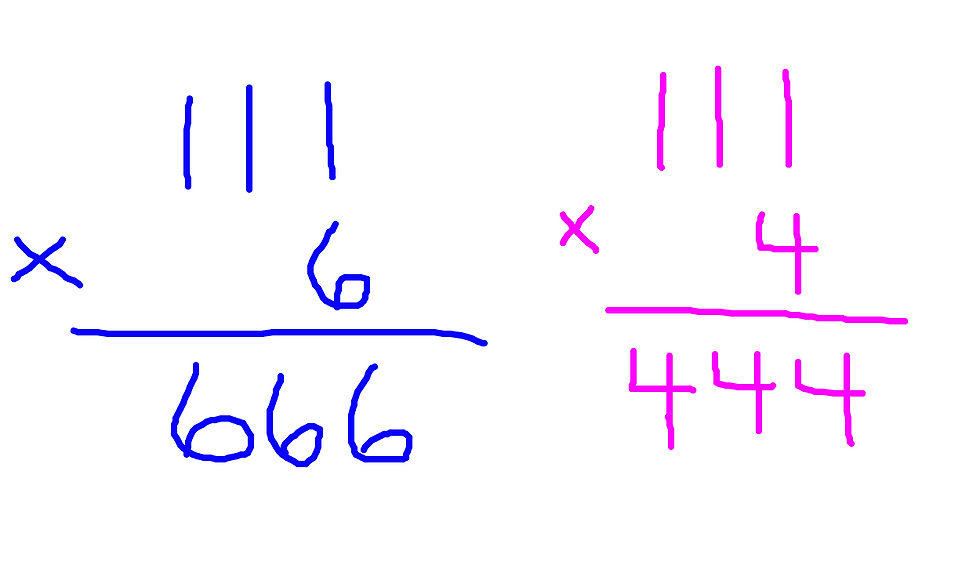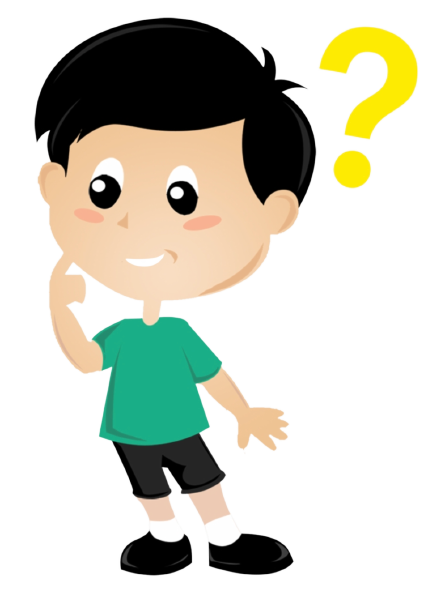top of page
• mmagocs4

# Fun Number Trick Magic to Engage Our Brains

I love magic! I admit it. I can watch a magician for hours and feel like a kid, mesmerized and wanting to know how the trick worked.Magic tricks are possible with math, too.

I love doing number tricks with students because I can tell their minds start to wonder. They become really interested in figuring out how to stump me or can't wait to do the trick to someone else. But I always want them to also try to understand why the trick works. Tricks can be a fun application of math rules.

I will go over one math trick today. It is a good trick for elementary and middle school students who know how to multiply multi-digit numbers.

###### Math TRICK Using Multiplication

To follow the steps to this trick, the person must know how to multiply multi-digit numbers. What does that mean? The person needs to know how to do problems such as 29 x 67 or 56 x 34, for example.

In this video, you'll see the four steps the "magician" asks someone to do (on the left) as well as each step of the trick after picking a number (on the right).

Math Trick with Number 37:

Perhaps as an adult you know why this works. Perhaps not. Either way, I encourage you to try the trick with your child a few times with different numbers. A pattern will emerge after it's performed a few times. See if it ignites a conversation or a curiosity to figure out why it works.

If you've given it some thought and you're wondering how it works, let me reveal the magician's secret:

Multiplication is like addition in that the order in which you add numbers together doesn't matter and the order in which you multiply numbers together also doesn't matter.

7 + 3 + 5 = 3 + 5 + 7 = 5 + 7 + 3 = 15

All of these are equal to 15 despite the different ordering of the numbers.

In the magic trick, I asked you to multiply your number by 3. Then, you took that number and multiplied it by 37. When I picked the number 6, it looked like this:

6 x 3 x 37 = 666

However, because of the commutative property of multiplication, the order of the numbers doesn't matter and I can rearrange the numbers into a different order and still get the same answer. So let's do that:

3 x 37 x 6 = 666

BUT WHY does it equal 666? Let's break it apart:

The first two numbers to get multiplied are 3 x 37. What is 3 x 37?

3 x 37 = 111

A-ha! 🤩 111 multiplied to any single-digit number between 1-9 will result in that digit repeating in the answer, such as 111 x 6 = 666. If I had chosen 4 to start with, it would have ended up being 111 x 4 because 3 x 37 = 111 and 111 x 4 = 444.Once the trick is understood, it's usually really fun for the child to be the magician and ask someone else to do the trick. Having the math knowledge can feel empowering!

After the trick, it's possible to spark some other math conversations:• Do you think there are other factors of 111?

• How would we be able to tell that 111 is divisible by 3? (a great example of divisibility rules)

• Name some other big numbers that we can be sure are divisible by 3.

• Is 111 a prime or composite number?

• Can any of the other operations use the commutative property? If so, which one/s?

Reach out with any questions at melissa@learnwithme123.com.

=+-=+-=+-=+-=+-=+-=+-=+-=+-=+-=+-=

Melissa Agocs

melissa@learnwithme123.com